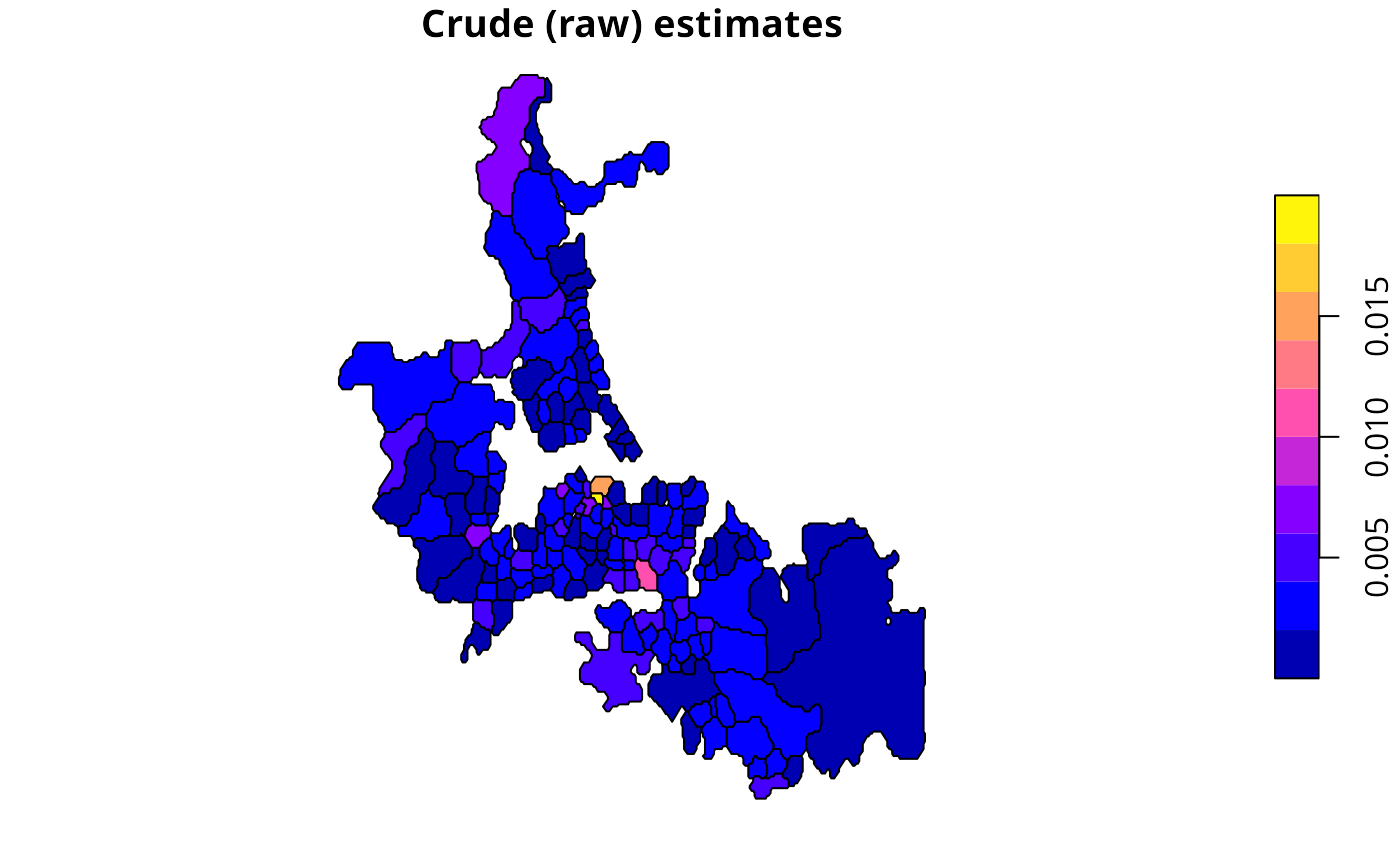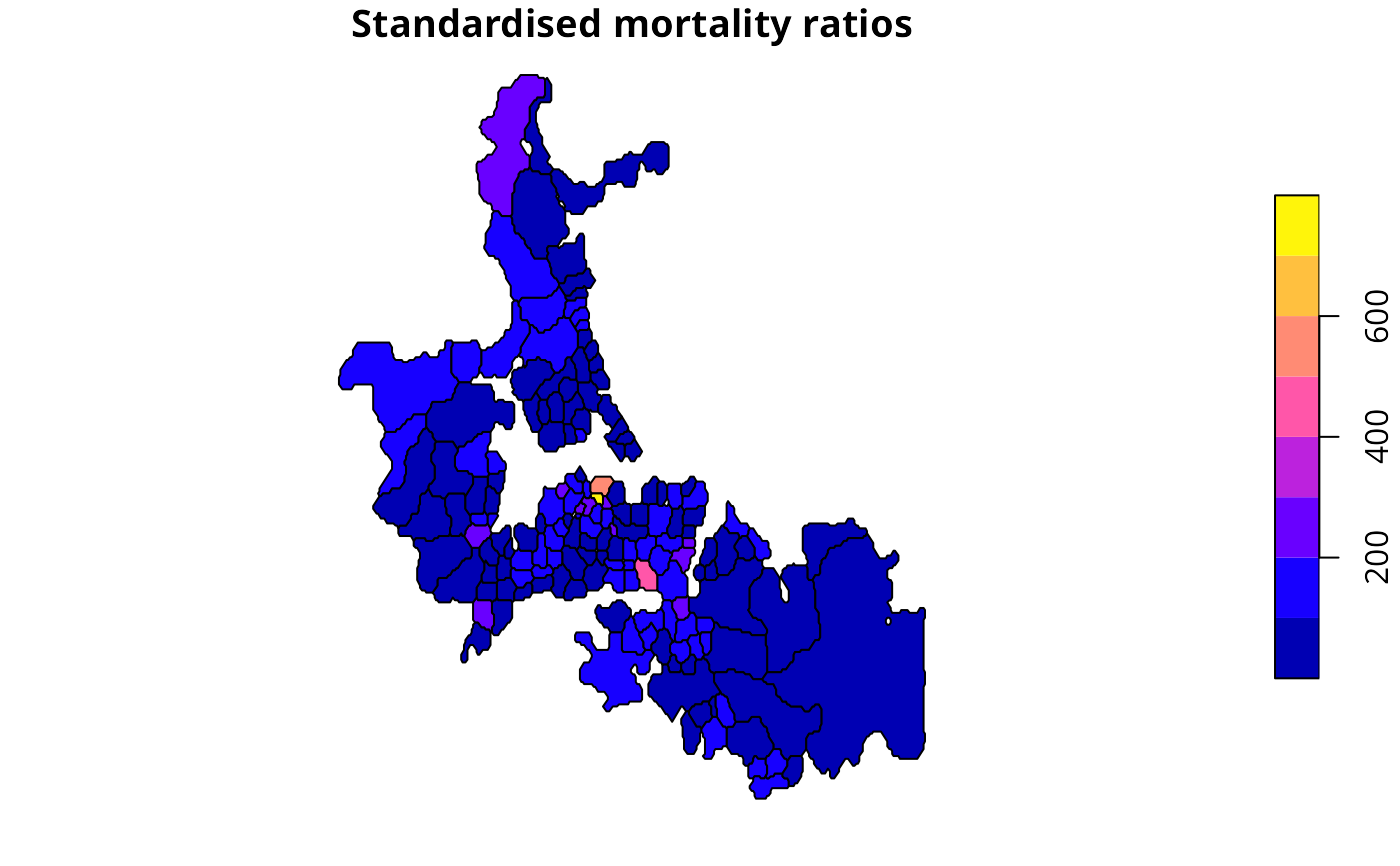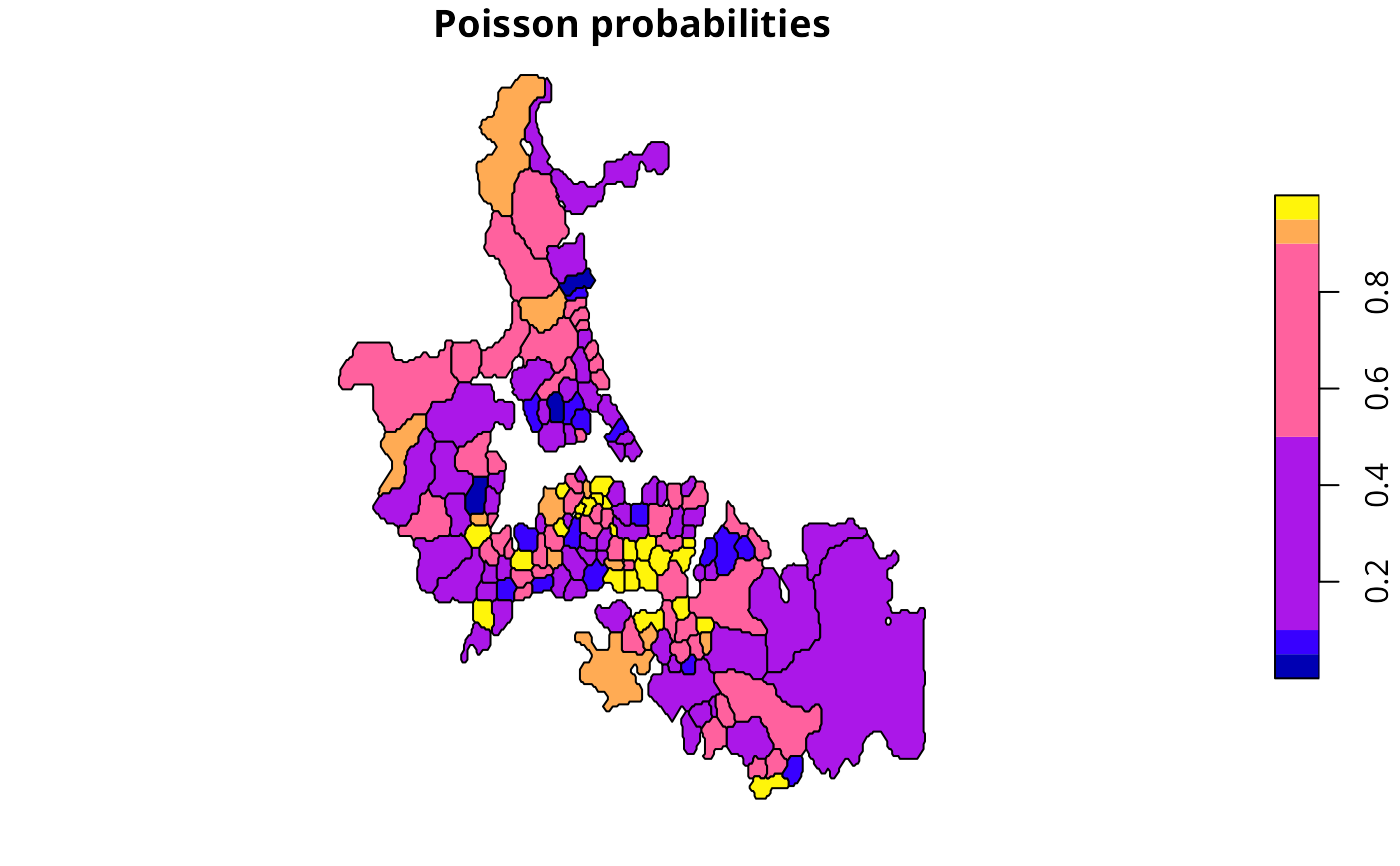The function returns a data frame of rates for counts in populations at risk with crude rates, expected counts of cases, relative risks, and Poisson probabilities.

probmap(n, x, row.names=NULL, alternative="less")

## Arguments

n

a numeric vector of counts of cases

x

a numeric vector of populations at risk

row.names

row names passed through to output data frame

alternative

default “less”, may be set to “greater”

## Details

The function returns a data frame, from which rates may be mapped after class intervals have been chosen. The class intervals used in the examples are mostly taken from the referenced source.

## Value

raw

raw (crude) rates

expCount

expected counts of cases assuming global rate

relRisk

relative risks: ratio of observed and expected counts of cases multiplied by 100

pmap

Poisson probability map values: probablility of getting a more extreme'' count than actually observed - one-tailed, default alternative observed “less” than expected

## Author

Roger Bivand Roger.Bivand@nhh.no

EBest, EBlocal, ppois

## Examples

auckland <- st_read(system.file("shapes/auckland.shp", package="spData"), quiet=TRUE)
res <- probmap(auckland$M77_85, 9*auckland$Und5_81)
rt <- sum(auckland$M77_85)/sum(9*auckland$Und5_81)
ppois_pmap <- numeric(length(auckland$Und5_81)) for (i in seq(along=ppois_pmap)) { ppois_pmap[i] <- poisson.test(auckland$M77_85[i], r=rt,
T=(9*auckland$Und5_81[i]), alternative="less")$p.value
all.equal(ppois_pmap, res$pmap) } res$id <- 1:nrow(res)
auckland$id <- res$id <- 1:nrow(res)
auckland_res <- merge(auckland, res, by="id")
plot(auckland_res[, "raw"], main="Crude (raw) estimates")plot(auckland_res[, "relRisk"], main="Standardised mortality ratios")plot(auckland_res[, "pmap"], main="Poisson probabilities",
breaks=c(0, 0.05, 0.1, 0.5, 0.9, 0.95, 1))